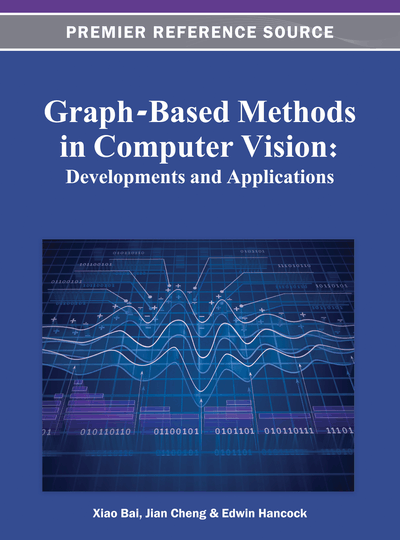# Normalized Projection and Graph Embedding via Angular Decomposition

Dengdi Sun (Anhui University, China), Chris Ding (Anhui University, China & University of Texas at Arlington, USA), Jin Tang (Anhui University, China) and Bin Luo (Anhui University, China)
DOI: 10.4018/978-1-4666-1891-6.ch012
Available
\$37.50
No Current Special Offers

## Abstract

Dimensionality reduction plays a vital role in pattern recognition. However, for normalized vector data, existing methods do not utilize the fact that the data is normalized. In this chapter, the authors propose to employ an Angular Decomposition of the normalized vector data which corresponds to embedding them on a unit surface. On graph data for similarity/kernel matrices with constant diagonal elements, the authors propose the Angular Decomposition of the similarity matrices which corresponds to embedding objects on a unit sphere. In these angular embeddings, the Euclidean distance is equivalent to the cosine similarity. Thus data structures best described in the cosine similarity and data structures best captured by the Euclidean distance can both be effectively detected in our angular embedding. The authors provide the theoretical analysis, derive the computational algorithm, and evaluate the angular embedding on several datasets. Experiments on data clustering demonstrate that the method can provide a more discriminative subspace.
Chapter Preview
Top

## Angular Decomposition

We start with a brief discussion of PCA, which is the most widely used dimensionality reduction method. Let the input data matrixcontains the collection of n data column vectors in p dimension space. In image processing, each column xi is a linearized array of pixels’ gray levels; in text processing, xi is a document. PCA finds the optimal low-dimensional (k-dim) subspace defined (spanned) by the principal directionsThe projected data points in the new subspace arePCA finds U and V by minimizing

(1)

## Complete Chapter List

Search this Book:
Reset